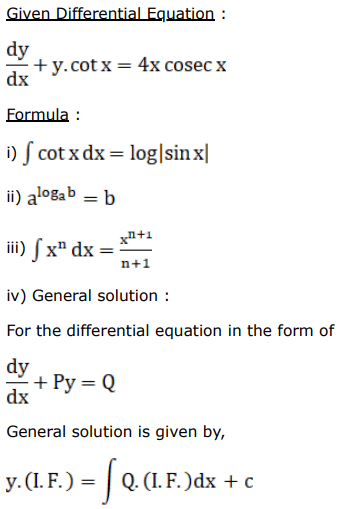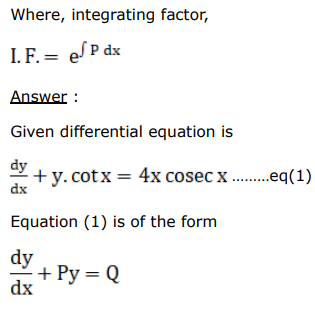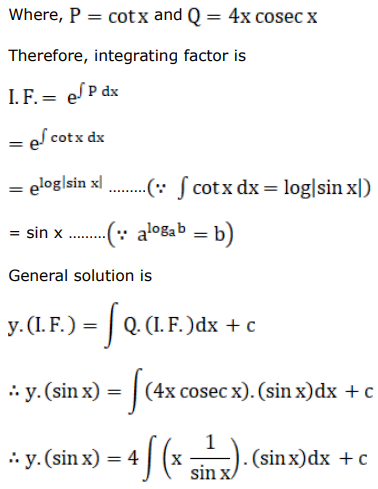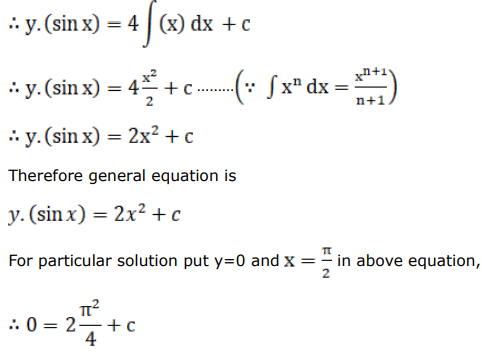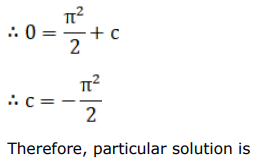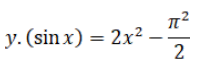# Find a particular solution satisfying the given condition for each of the following differential equations.

Question:

Find a particular solution satisfying the given condition for each of the following differential equations.

$\frac{d y}{d x}+y \cot x=4 x \cos e c x$, given that $y=0$ when $x=\frac{\pi}{2}$.

Solution: